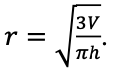# MA.912.AR.7.1Export Print
Solve one-variable radical equations. Interpret solutions as viable in terms of context and identify any extraneous solutions.
General Information
Subject Area: Mathematics (B.E.S.T.)
Strand: Algebraic Reasoning
Status: State Board Approved

## Benchmark Instructional Guide

### Terms from the K-12 Glossary

• Domain
• Intercept
• Inverse functions

### Vertical Alignment

Previous Benchmarks

Next Benchmarks

### Purpose and Instructional Strategies

In Algebra I, students solved one variable equations including linear, quadratic and absolute value, and determined if the solution(s) was viable. In Math for College Algebra, students solve one variable equations involving radicals.
• Instruction for solving radical equations will follow many of the same characteristics. One will still need to isolate the one variable you have in order to solve. Students will need to understand the inverse relationship between radicals and roots as well as how to convert between radical form and rational exponent form (MTR.2.1)
• Students will also need to understand laws of exponents and how to utilize them to simplify radical expressions and solve radical equations. Students should feel comfortable finding and representing the solutions to a radical equation algebraically, graphically, and numerically (with a table) (MTR.2.1)
• Students should be able to determine if their solution is viable using multiple representations. Students should also be able to explain how many solutions a radical equation will give them as well as what features of that radical equation will determine how many solutions it has as well as if there are any extraneous solutions (MTR.6.1).

### Common Misconceptions or Errors

• Students may not understand how to determine if a solution is extraneous.
• Teachers should present the students with a few examples when extraneous solutions arise and ask the students to look for patterns for when those extraneous solutions arise. Teachers should remind students that solving an equation is finding the values of the variable that make the statement true.
• Students may not completely understand the relationships between roots and radicals so they may use the wrong root or exponent to isolate the variable when solving the equation.
• Student may square an individual term instead of squaring the whole side of the equation when trying to solve a radical equation.
• For example, in the equation $\sqrt{x}$$\sqrt{x + 2}$ = 2, students may square the left term on the left side of the equation and not realize to square whole expression on the left side.
• Students may not understand how to use a graph or table to justify what they have found algebraically.

• Part A. Create a one variable radical equation that would have one solution.
• Part B. Explain what would you have to change about the equation to have two solutions.
• Part C. Explain what would you have to change about the equation to have no solution.

• An equation is given.
$\sqrt{4x - 1}$ = 3
• Part A. What is/are the solution(s)?
• Part B. Describe how would you find the solution(s) on a graph.
• Part C. Describe how would you find the solution(s) in a table.

• Part A. Explain what it means to get an extraneous solution to a radical equation.
• Part B. What features will happen in a radical equation to make an extraneous solution arise?
• Part C. Give an example of a radical equation with an extraneous solution.

• One estimate for how far one can see on a clear day is given by the formula $v$ = 1.225$\sqrt{\alpha }$, where $v$ represents the visibility in miles and α represents the altitude in feet.
• Part A. A person is parasailing and can see 64 kilometers to the horizon, use the given formula to determine how far above the ground the person parasailing.
• Part B. If you were given a value for the altitude rather than the visibility, how would that change our approach to finding a solution? What would the value for α mean in the context?

### Instructional Items

Instructional Item 1
• Find the solution(s) to the following equation $\sqrt{x - 3}$ = −5. Explain how you found the solution(s).

Instructional Item 2
• Find all of the solution(s) to the following equation $\sqrt{x}$ =  $\sqrt{x}$

Instructional Item 3
• Which of these equations has an extraneous solution?
a. $\sqrt{2 - x}$ = $x$
b. $\sqrt{5 - x}$ = 2
c. $\sqrt{x}$ − 12 = 0
d. $\frac{\text{4x}}{\text{6}}$ = $x$

Instructional Item 4
• The formula for a cone of a cone written in terms of the radius is• Part A. A mound of mulch is piled in the shape of a cone with the height equal to three times the radius. Calculate the volume of such a mound of mulch whose radius equals 2.5 yards.
• Part B. Is your solution that you found viable? How do you know?

*The strategies, tasks and items included in the B1G-M are examples and should not be considered comprehensive.

## Related Courses

This benchmark is part of these courses.
1200330: Algebra 2 (Specifically in versions: 2014 - 2015, 2015 - 2022, 2022 and beyond (current))
1200340: Algebra 2 Honors (Specifically in versions: 2014 - 2015, 2015 - 2022, 2022 and beyond (current))
1200400: Foundational Skills in Mathematics 9-12 (Specifically in versions: 2014 - 2015, 2015 - 2022, 2022 and beyond (current))
7912095: Access Algebra 2 (Specifically in versions: 2016 - 2018, 2018 - 2019, 2019 - 2022, 2022 and beyond (current))
1200710: Mathematics for College Algebra (Specifically in versions: 2022 and beyond (current))

## Related Access Points

Alternate version of this benchmark for students with significant cognitive disabilities.
MA.912.AR.7.AP.1: Solve one-variable radical equations and identify any extraneous solutions.

## Related Resources

Vetted resources educators can use to teach the concepts and skills in this benchmark.